SBOS673D September   2017  – December 2018

PRODUCTION DATA.

1. Features
2. Applications
3. Description
4. Revision History
5. Pin Configuration and Functions
6. Specifications
7. Detailed Description
1. 7.1 Overview
2. 7.2 Functional Block Diagrams
3. 7.3 Feature Description
4. 7.4 Device Functional Modes
8. Application and Implementation
1. 8.1 Application Information
2. 8.2 Typical Applications
1. 8.2.1 Active Filters
2. 8.2.2 Implementing a 2:1 Active Multiplexer
3. 8.2.3 1-Bit PGA Operation
9. Power Supply Recommendations
10. 10Layout
11. 11Device and Documentation Support
12. 12Mechanical, Packaging, and Orderable Information

• DCK|5
• DBV|6
• DCK|5

6.10 Typical Characteristics: ±2.5-V to ±1.5-V Split Supply

with PD = VCC and TA ≈ 25°C (unless otherwise noted)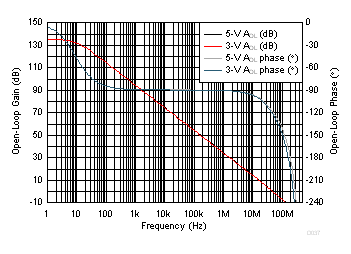Figure 37. Open-Loop Gain and Phase vs Frequency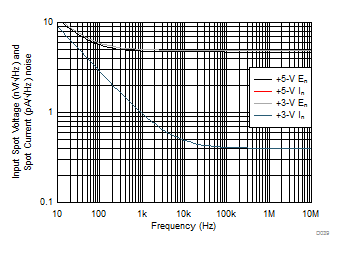Measured then fit to ideal 1/f model
Figure 39. Input Spot Noise Density vs Frequency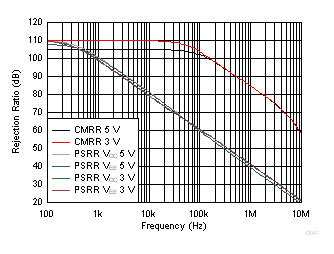Simulated curves
Figure 41. CMRR and PSRR vs Frequency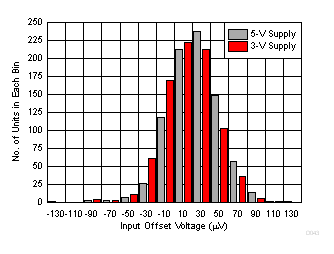830 units at each supply voltage
Figure 43. Input Offset Voltage Distribution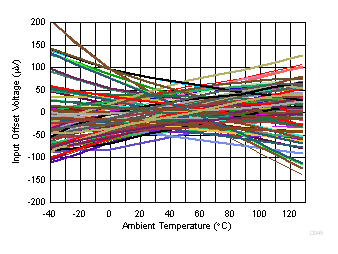50 units at 5-V and 3-V supply
Figure 45. Input Offset Voltage vs Ambient Temperature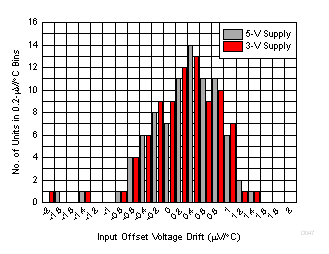–40°C to +125°C fit, 82 units, DBV package
Figure 47. Input Offset Voltage Drift Distribution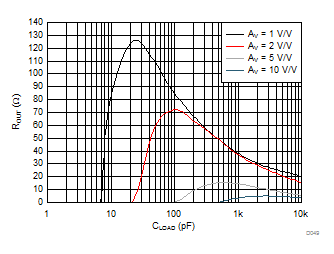See Figure 66 and Table 2, small signal, targeting 30° phase margin
Figure 49. Output Resistor vs CLOAD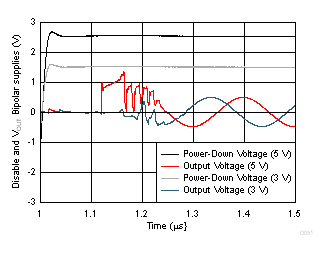Figure 51. Turn-On Time to Sinusoidal Input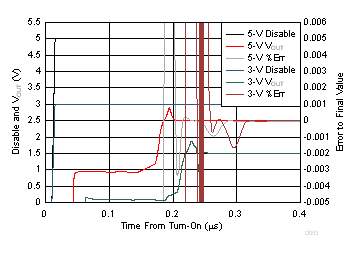Figure 53. Gain of 1 Turn-On Time to Final DC Value at Midscale (Simulated)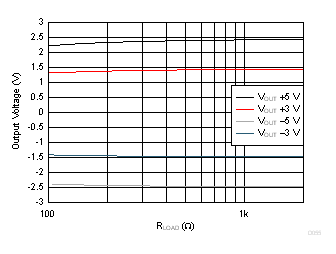Figure 55. Output Voltage Swing vs Load Resistor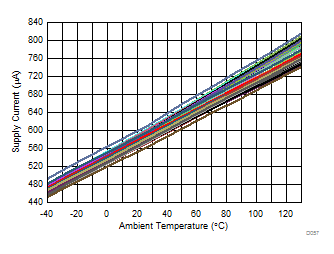50 units at 5-V and 3-V supply
Figure 57. Supply Current vs Ambient Temperature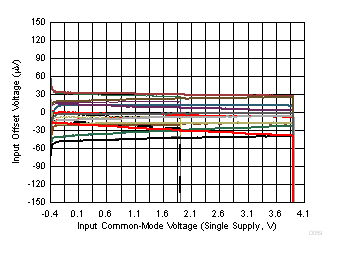12 units, 5-V and 3-V supplies
Figure 59. Input Offset Voltage vs
Input Common-Mode VoltageCrosstalk for the OPA2837 only
Figure 61. Crosstalk vs Frequency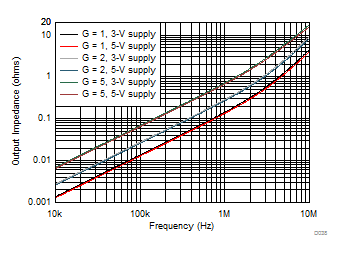Figure 74 and Table 2 (simulation)
Figure 38. Closed-Loop Output Impedance vs Frequency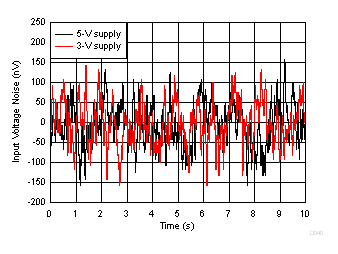Input-referred voltage noise RS = 0 Ω
Figure 40. Low-Frequency Voltage Noise vs Time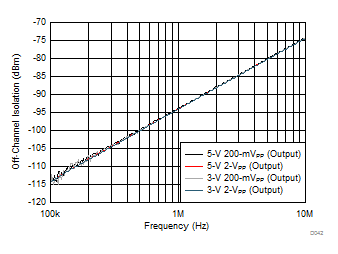Figure 42. Disabled Isolation Noninverting Input to Output vs Frequency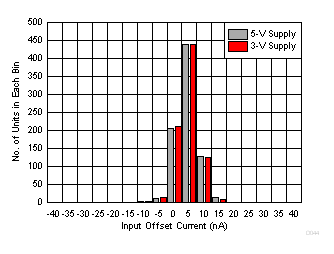830 units at each supply voltage
Figure 44. Input Offset Current Distribution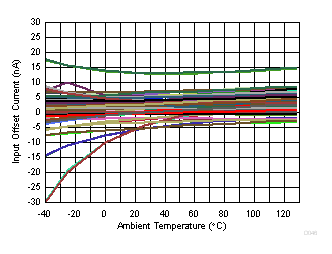50 units at 5-V and 3-V supply
Figure 46. Input Offset Current vs Ambient Temperature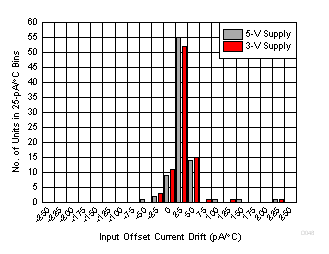–40°C to +125°C fit, 82 units, DBV package
Figure 48. Input Offset Current Drift Distribution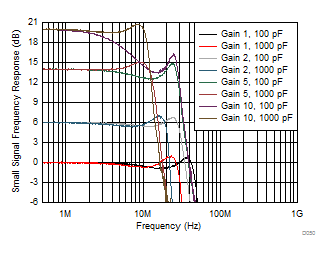Figure 50. Small-Signal Frequency Response vs CLOAD
With Recommended ROUT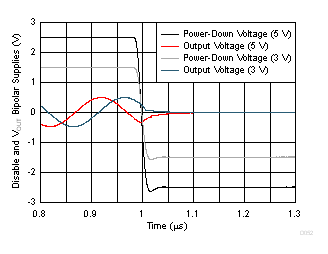Figure 52. Turn-Off Time to Sinusoidal Input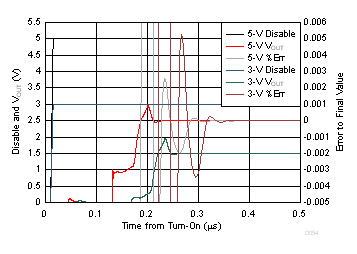Figure 54. Gain of 2 Turn-On Time to Final DC Value at Midscale (Simulated)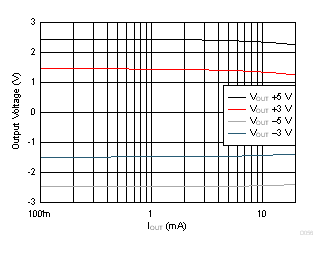Figure 56. Output Saturation Voltage vs Load Current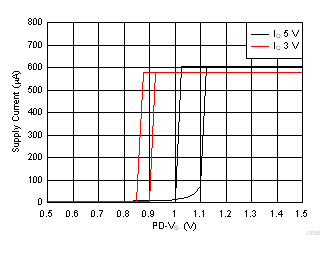Figure 58. Supply Current vs Power-Down Voltage
(Turn-On Higher Than Turn-Off)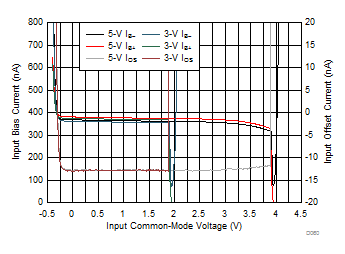Measured single device, 5-V and 3-V supplies
Figure 60. Input Bias and Offset Current vs VICM